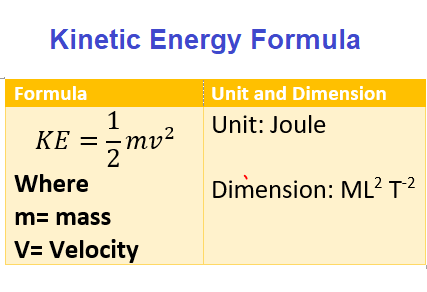# Kinetic Energy Formula, Definition,Concepts, examples

## (5) Kinetic energy Definition and Formula

• Kinetic energy is the energy possessed by the body by virtue of its motion
• Body moving with greater velocity would posses greater K.E(Kinetic energy) in comparison of the body moving with slower velocity
• Consider a body of mass m moving under the influence of constant Force F.From newton's second law of motion
$F=ma$
Where a is the acceleration of the body
• If due to this acceleration a,velocity of the body increases from v1 to v2 during the displacement d then from equation of motion with constant acceleration, we have
$v_2^2 -v_1^2=2ad$ or
$a= \frac {v_2^2 -v_1^2}{2d}$ Using this acceleration in Newton's second law of motion
we have
$F=m\frac {v_2^2 -v_1^2}{2d}$
or
$Fd=\frac {m(v_2^2 -v_1^2)}{2}$
or
$Fd=\frac {1}{2}mv_2^2 -\frac {1}{2}mv_1^2$           (7)
We know that Fd is the work done by the force F in moving body through distance d
• In equation(7),quantity on the right hand side $\frac {1}{2}mv^2$ is called the kinetic energy of the body
Thus
$Kinetic \; energy =\frac {1}{2}mv^2$
• Finally we can define Kinetic Energy of the body as one half of the product of mass of the body and the square of its Speed
• Thus we see that quantity (mv2/2) arises purely because of the motion of the body
• In equation 7 quantity
K2=mv22/2
is the final Kinetic energy of the body and
K1=mv12/2
is the initial Kinetic of the body .Thus equation 7 becomes
W=K2-K1=ΔK           (9)
• Where ΔK is the change in KE.Hence from equation (9) ,we see that work done by a force on a body is equal to the change in kinetic energy of the body
• Kinetic energy like work is a scalar quantity
• Unit of KE is same as that of work i.e Joule
• Since velocity is a vector quantity, we can expressed Kinetic energy as $K=\frac {1}{2}m \mathbf{v}. \mathbf{v}$
Here $\mathbf{v}. \mathbf{v}$ is the scalar dot productExample-1
Calculate the Kinetic energy of the object of mass 10 kg and velocity 5 m/s?
Solution
$Kinetic \; energy =\frac {1}{2}mv^2$

$K = \frac {1}{2} 10 \times 5^2 = 50 J$

Example-2
Calculate the Kinetic energy of the object of mass 10kg and velocity v = (2i + 3j) m/s at any instant?
Solution
$K=\frac {1}{2}m \mathbf{v}. \mathbf{v}$

$K= \frac {1}{2} \times 10 \times (2^2 + 3^2) =65 J$
Check out our Kinetic energy Calculator